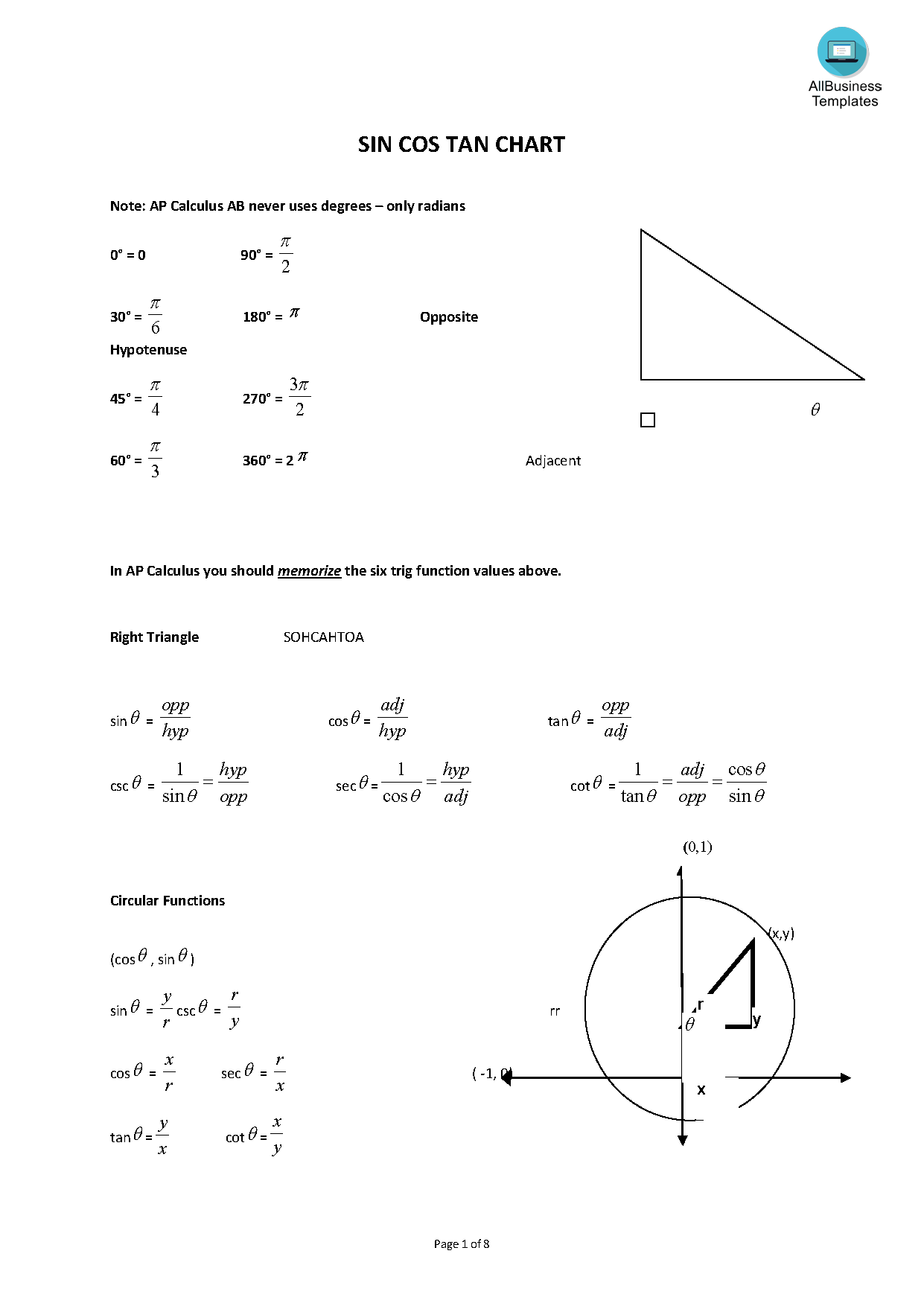# Sin Cos Tan Charti-click ang larawan para lumaki / i-click ang buton sa ibaba para makita ang marami pang larawan

What is the rule for Sin Cos Tan?  How to calculate the trigonometric functions, sine, cosine, and tangent?

In mathematics, the trigonometric functions (also called circular functions, angle functions, or goniometric functions are functions of an angle. They relate the angles of a triangle to the lengths of its sides. Trigonometric functions are important in the study of triangles and modeling periodic phenomena, among many other applications.

The most familiar trigonometric functions are the sine, cosine, and tangent. In this Excel worksheet, you can easily calculate the sine, cosine, and tangent. In the context of the standard unit circle (a circle with radius 1 unit), where a triangle is formed by a ray starting at the origin and making some angle with the x-axis, the sine of the angle gives the length of the y-component (the opposite to the angle or the rise) of the triangle, the cosine gives the length of the x-component (the adjacent of the angle or the run), and the tangent function gives the slope (y-component divided by the x-component).

Mathematicians regularly need spreadsheets, worksheets, or forms, and often more than any other industry. Therefore, we support you by providing this A sin (Bx+c) Excel template, which will save you time, cost, and effort and help you to reach the next level of success.

This blank A sin (Bx+c) is intuitive, ready-to-use, and structured in a smart way. Try it now and let this mathematics template inspire you. We certainly encourage you to use this Sin Cos Tan Chart for your own good and are confident it will fit your needs

DISCLAIMER
Wala sa 'site' na ito ang dapat ituring na legal na payo at walang abogado-kliyenteng relasyon na itinatag.

Mag-iwan ng tugon. Kung mayroon kang anumang mga katanungan o mga komento, maaari mong ilagay ang mga ito sa ibaba.### Pinakabagong paksa

Iba pang mga paksa